# The Slope of a Line Slope Slope rate

• Slides: 17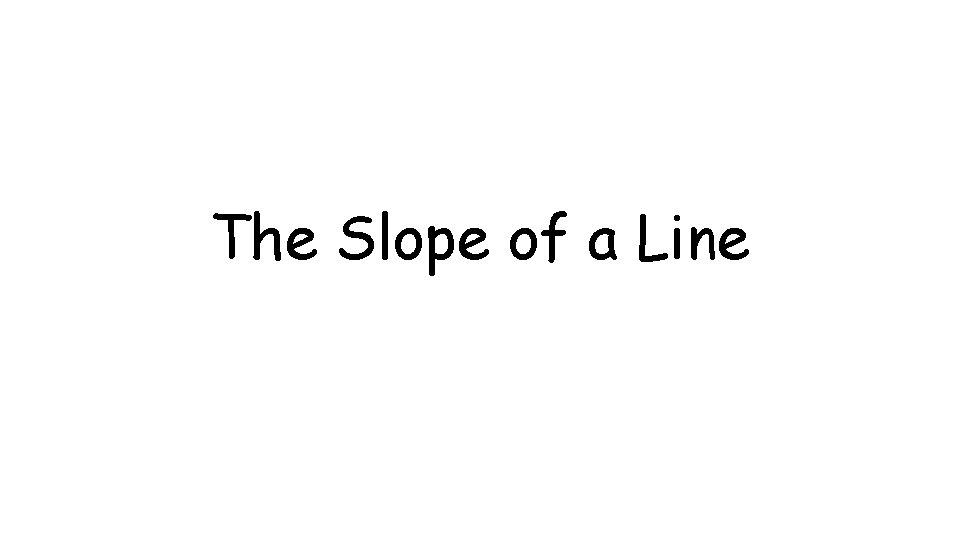The Slope of a LineSlope • Slope: rate of change of a line • The steepness of a line y (x 2, y 2) y 2 – y 1 rise (x 1, y 1) • Where m = slope x 2 – x 1 run x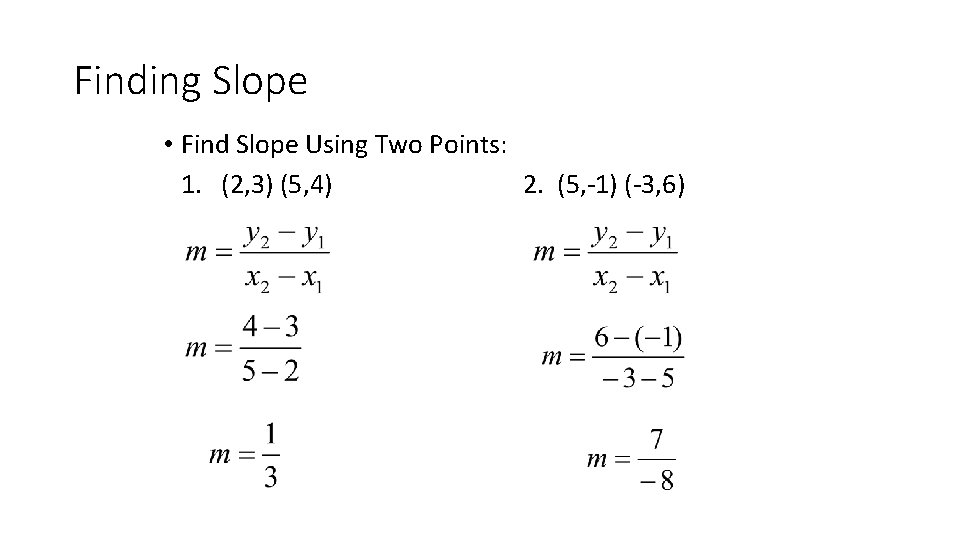Finding Slope • Find Slope Using Two Points: 1. (2, 3) (5, 4) 2. (5, -1) (-3, 6)Positive Slope • A line that rises from left to right is a positive slope.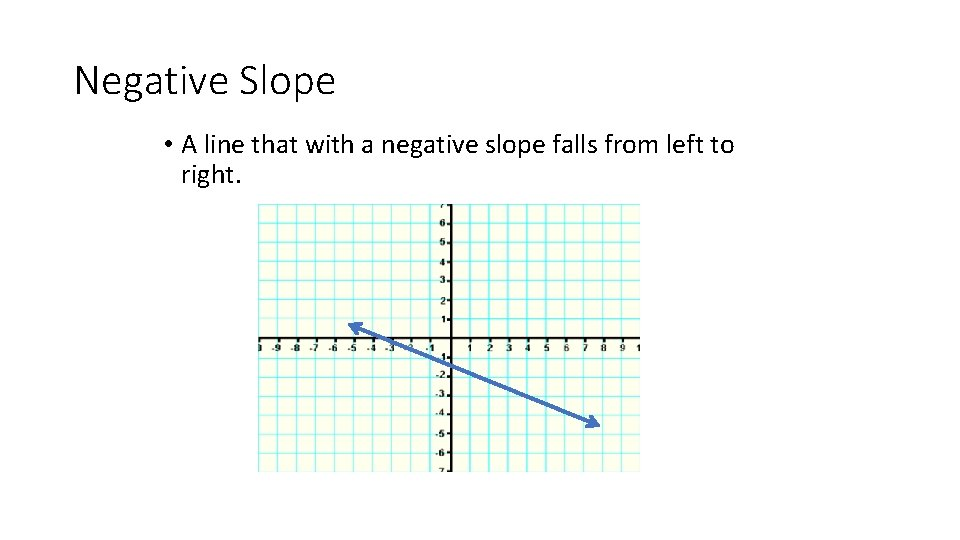Negative Slope • A line that with a negative slope falls from left to right.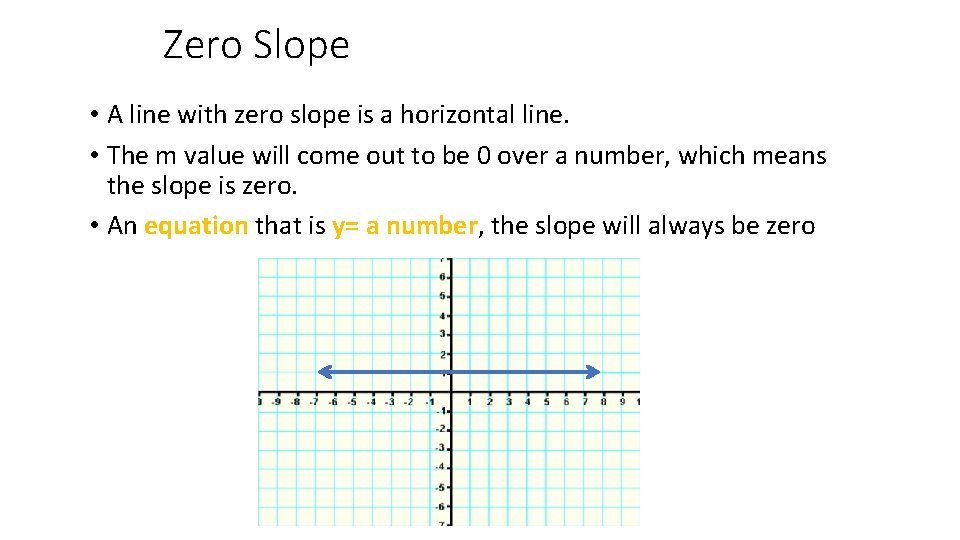Zero Slope • A line with zero slope is a horizontal line. • The m value will come out to be 0 over a number, which means the slope is zero. • An equation that is y= a number, the slope will always be zeroUndefined Slope • A line that is an undefined slope is a vertical line. • The m value will come out to be a non-zero number over 0. • An equation that is x= a number will always have an undefined slopeWriting Linear EquationsSlope-Intercept When Given a Table When given table • +1 +1 x 0 1 2 y 2 4 6 +2 +2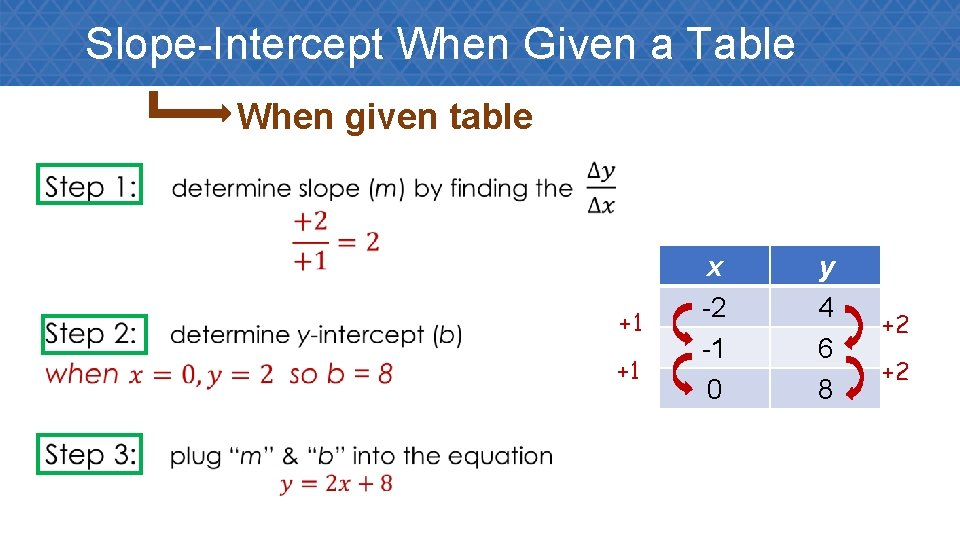Slope-Intercept When Given a Table When given table • +1 +1 x -2 -1 0 y 4 6 8 +2 +2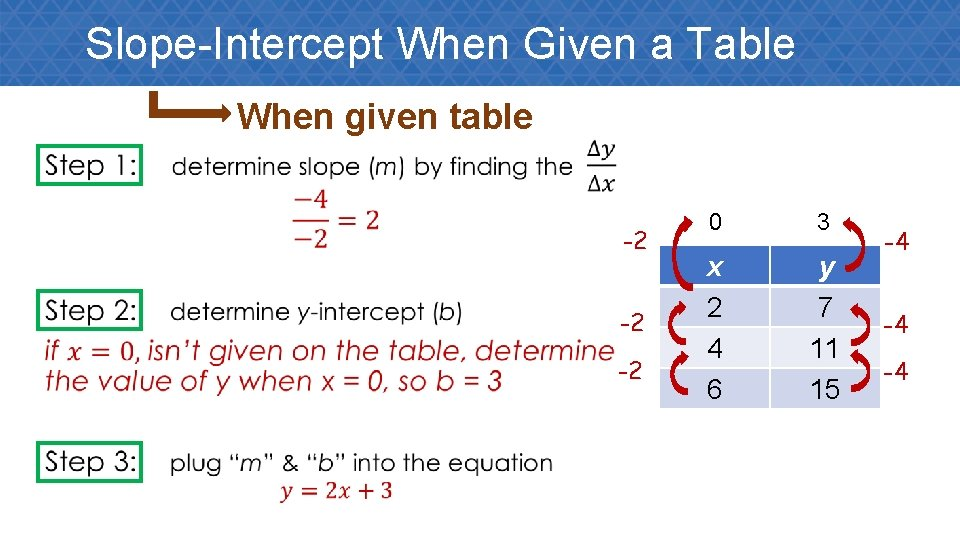Slope-Intercept When Given a Table When given table • -2 -2 -2 0 3 x 2 4 6 y 7 11 15 -4 -4 -4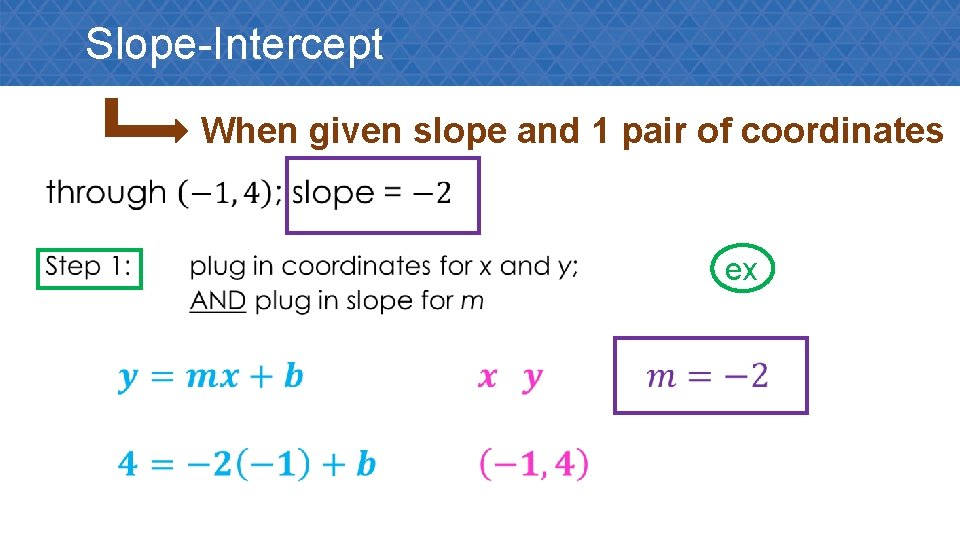Slope-Intercept When given slope and 1 pair of coordinates • exSlope-Intercept When given slope and 1 pair of coordinates •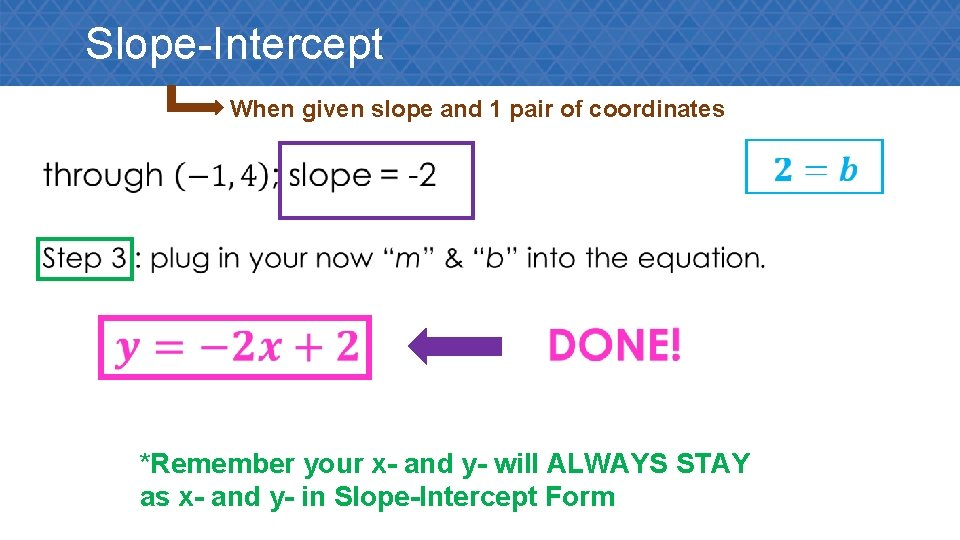Slope-Intercept When given slope and 1 pair of coordinates • *Remember your x- and y- will ALWAYS STAY as x- and y- in Slope-Intercept Form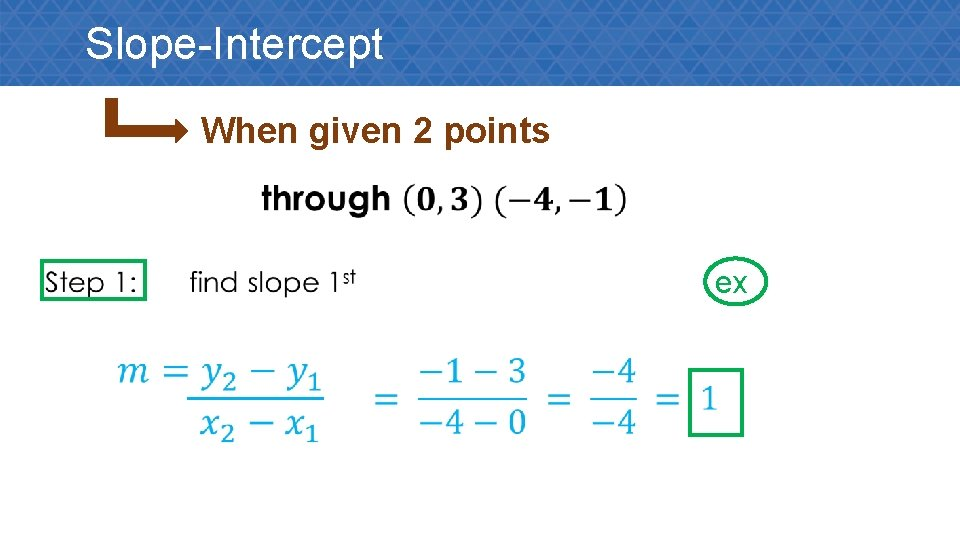Slope-Intercept When given 2 points • ex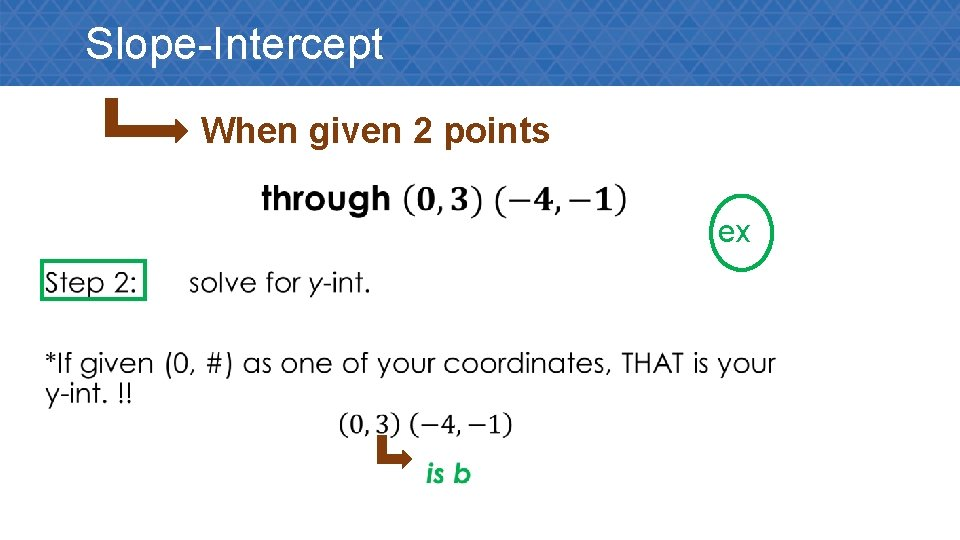Slope-Intercept When given 2 points • ex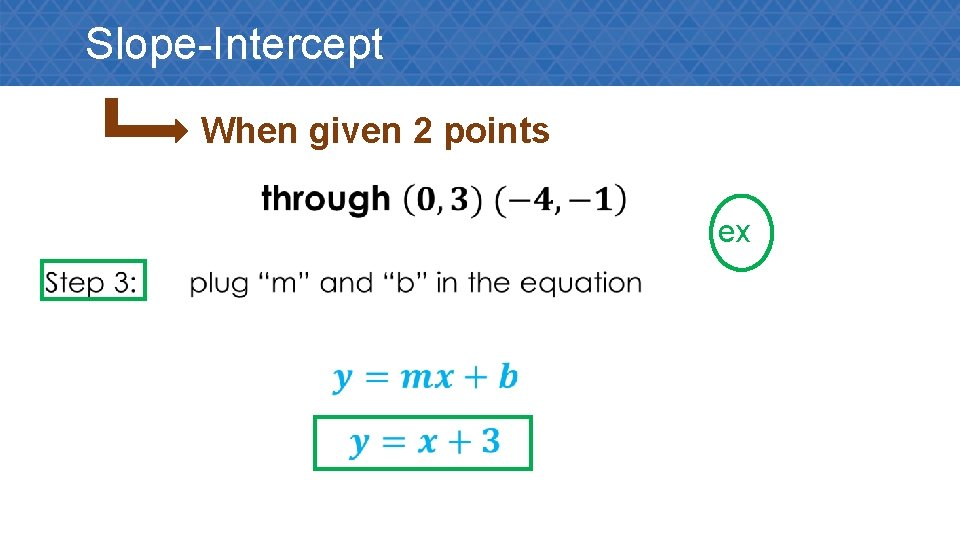Slope-Intercept When given 2 points • ex